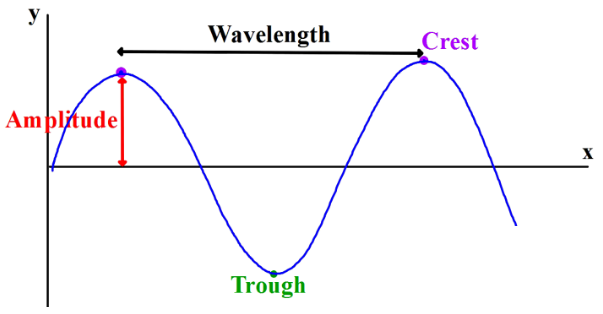# Terms used in Wave Motion

Some of the common terms used in wave motion are as follows.Displacement: The distance traveled by the wave wave form the mean position at any instant of time is called its displacement. It is represented by y.

Wavelength: It is defined as the distance between two successive crest and trough or compression or rarefraction.

Frequency: The total number of oscillation made by the particle in one second is called frequency. It is denoted by f. Frequency and time as inverse relation between them.

Period: The time taken by the vibrating particle in the medium to complete one oscillation is called period. It is denoted by T.

Wave velocity: The distance traveled by the wave in one second is called wave velocity.
i.e., wave velocity = distance traveled by the wave / time taken.

or, v = λ/T = λ × 1/f = λf

∴ v = λf

Phase: The state of motion of a particle at a given place and time is called phase and the angle made by the vibrating particle is called phase angle .

Do you like this article ? If yes then like otherwise dislike : 2

#### One Response to “Terms used in Wave Motion”

1.s

error in λ × 1/f = λf
correct as λ / 1/f = λf